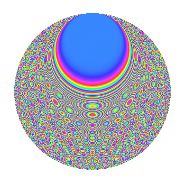# Properties

 Label 806.2.cbLevel 806 Weight 2 Character orbit cb Rep. character $$\chi_{806}(137,\cdot)$$ Character field $$\Q(\zeta_{60})$$ Dimension 608 Newforms 1 Sturm bound 224 Trace bound 0

# Related objects

## Defining parameters

 Level: $$N$$ = $$806 = 2 \cdot 13 \cdot 31$$ Weight: $$k$$ = $$2$$ Character orbit: $$[\chi]$$ = 806.cb (of order $$60$$ and degree $$16$$) Character conductor: $$\operatorname{cond}(\chi)$$ = $$403$$ Character field: $$\Q(\zeta_{60})$$ Newforms: $$1$$ Sturm bound: $$224$$ Trace bound: $$0$$

## Dimensions

The following table gives the dimensions of various subspaces of $$M_{2}(806, [\chi])$$.

Total New Old
Modular forms 1856 608 1248
Cusp forms 1728 608 1120
Eisenstein series 128 0 128

## Trace form

 $$608q - 12q^{7} - 80q^{9} + O(q^{10})$$ $$608q - 12q^{7} - 80q^{9} + 8q^{11} - 4q^{12} + 20q^{13} - 24q^{14} - 76q^{16} + 24q^{17} + 32q^{18} + 4q^{19} - 112q^{21} - 12q^{22} + 60q^{27} + 32q^{28} - 12q^{29} - 16q^{31} + 92q^{33} - 56q^{34} + 4q^{35} - 4q^{37} + 108q^{38} - 120q^{39} + 8q^{41} - 48q^{43} - 48q^{45} - 64q^{46} + 8q^{47} + 24q^{51} + 4q^{52} + 52q^{53} - 148q^{57} - 12q^{58} - 4q^{59} - 12q^{61} + 36q^{62} - 204q^{63} + 128q^{65} - 32q^{66} + 52q^{67} - 180q^{69} - 48q^{70} - 8q^{71} - 32q^{72} - 4q^{73} + 116q^{75} + 52q^{76} - 144q^{77} - 92q^{78} + 28q^{79} + 220q^{81} - 56q^{83} - 48q^{84} - 104q^{85} - 12q^{86} - 32q^{87} + 128q^{89} - 104q^{91} - 172q^{93} + 8q^{94} + 84q^{97} - 72q^{98} - 240q^{99} + O(q^{100})$$

## Decomposition of $$S_{2}^{\mathrm{new}}(806, [\chi])$$ into irreducible Hecke orbits

Label Dim. $$A$$ Field CM Traces $q$-expansion
$$a_2$$ $$a_3$$ $$a_5$$ $$a_7$$
806.2.cb.a $$608$$ $$6.436$$ None $$0$$ $$0$$ $$0$$ $$-12$$

## Decomposition of $$S_{2}^{\mathrm{old}}(806, [\chi])$$ into lower level spaces

$$S_{2}^{\mathrm{old}}(806, [\chi]) \cong$$ $$S_{2}^{\mathrm{new}}(403, [\chi])$$$$^{\oplus 2}$$